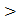# For the circuit showing below, the current through the Zener diode is:(a) 5 mA (b) Zero (c) 14 mA (d) 9 mA

## Question ID - 50280 :- For the circuit showing below, the current through the Zener diode is:(a) 5 mA (b) Zero (c) 14 mA (d) 9 mA

3537

Assuming Zener diode does not undergo

Breakdown, current in circuit==8mA

∴voltage drop across diode=80 V50 V. The diode undergo breakdown.Current is R1==14mA

Current is R2==5 mA

∴Current through diode=9mA

Next Question :
 Four equal point charges Q each are placed in the xy placed at (0, 2), (4, 2), (4, −2) and (0, −2). The work required to put a fifth charge Q at the origin of the coordinate system will be: (a)(b)(c)(d)